# Finding the work done by Spiderman

ChiralSuperfields
Homework Statement:
Relevant Equations:
For this problem,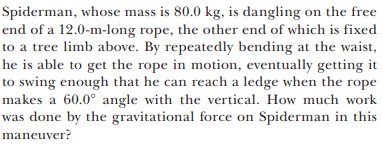The answer is ##-4.70 kJ##. I am not sure what I am doing wrong.

My working is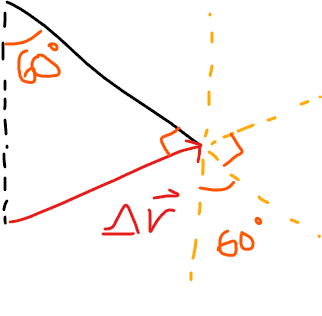## W = mgr\cos\theta ##
## W = mgr\cos150 ## (since angle between ##\vec g## and ##\vec r## is 150 degrees)
## W = -mgr\frac {\sqrt{3}}{2} ##
## W = -mgr\frac {\sqrt{3}}{2} ##
## W = (-80)(9.81)(12\sin60)(\frac {\sqrt{3}}{2}) ##
## W = -7063.2 J ##

Would some please be to offer some guidance?

Many thanks!

Homework Helper
Gold Member
2022 Award
## W = mgr\cos\theta ##
Justify that.

•ChiralSuperfields
Homework Helper
Gold Member

#### Attachments

• Spiderman.pdf
13.7 KB · Views: 13
•ChiralSuperfields
ChiralSuperfields
Thank you for your replies @haruspex and @Lnewqban!

##W = \vec F \times \vec r ##
## W = Fr\cos\theta ## from definition of dot product
F = mg since we are finding work done gravity
##W = mgr\cos\theta ##

Many thanks!

•Lnewqban
Homework Helper
Gold Member
2022 Award
##W = \vec F \times \vec r ##
No, that’s the cross product. You want the dot product.
But leaving that aside, what relationship does ##\vec r## need to have to the force in order for the result to be work done by the force?

•ChiralSuperfields
ChiralSuperfields
No, that’s the cross product. You want the dot product.
But leaving that aside, what relationship does ##\vec r## need to have to the force in order for the result to be work done by the force?
Sorry, yeah I forgot to do the dot product.

##\vec r## can be at any angle expect ##\theta = 90, 270, 450, n + 180## with the force

Many thanks!

Homework Helper
Gold Member
2022 Award
I suggest you redo the problem with 1 degree rather than 60 degrees and you should see your mistake.

•MatinSAR and ChiralSuperfields
Homework Helper
Gold Member
2022 Award
##\vec r## can be at any angle expect ##\theta = 90, 270, 450, n + 180## with the force
No, I mean the functional relationship. "##\vec r## is the displacement through which …".

•ChiralSuperfields
ChiralSuperfields
I suggest you redo the problem with 1 degree rather than 60 degrees and you should see your mistake.
Thank you @PeroK! I have tried that now. I got a number much under the answer now.

Many thanks!

ChiralSuperfields
No, I mean the functional relationship. "##\vec r## is the displacement through which …".
Thank you @haruspex! Spider man moves?

Thank you !

Homework Helper
Gold Member
2022 Award
Thank you @haruspex! Spider man moves?

Thank you !
Wait, I'm sorry - I have objected to the wrong line.
It's this one:
## W = mgr\cos150 ## (since angle between ##\vec g## and ##\vec r## is 150

•ChiralSuperfields and MatinSAR
Homework Helper
Gold Member
Thank you for your replies @haruspex and @Lnewqban!

##W = \vec F \times \vec r ##
## W = Fr\cos\theta ## from definition of dot product
F = mg since we are finding work done gravity
##W = mgr\cos\theta ##

Many thanks!
Who is F and who is W in your diagram?

The weight of Spiderman, force which has done some work, has a unique direction of action: vertical.

•ChiralSuperfields
ChiralSuperfields
Thank you for your replies @haruspex and @Lnewqban!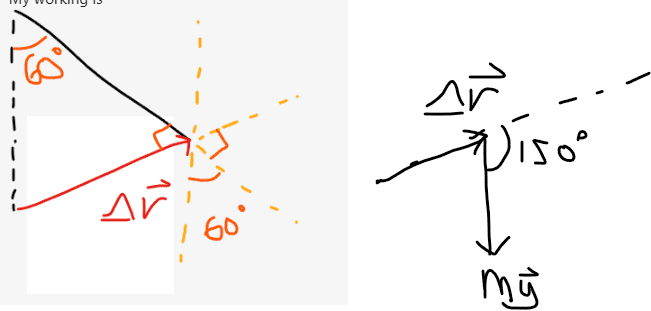Sorry I don't understand how the angle between the line extending from the displacement vector and the force of gravity is not 150 degrees.

I think I must have got the angles wrong or something in the diagram. Could someone please show me how to correctly calculate the angle between the displacement and weight vector?

F is the force of gravity acting on spider man and W is the work done by the force of gravity on the spider man.

Many thanks!

Homework Helper
You have a triangle with two equal sides (length of the wire in the two positions) and 60 degree angle at the top. What are the other two angles?

•ChiralSuperfields
ChiralSuperfields
You have a triangle with two equal sides (length of the wire in the two positions) and 60 degree angle at the top. What are the other two angles?

I did not see that it was an isosceles triangle! The two other angles will be 60 degrees each.

I will redo my diagram now.

Many thanks!

Homework Helper
Actually is equilateral, isn't it?

•ChiralSuperfields
ChiralSuperfields
Actually is equilateral, isn't it?

True, you are right! I did not know that an equilateral triangle is a special case of an isosceles triangle!

Many thanks!

ChiralSuperfields
Here is the new diagram with the equilateral triangle: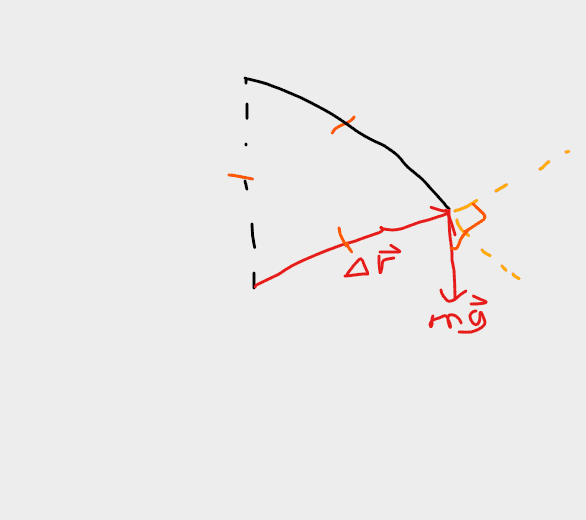It still seems to give ##\theta = 150## thought. I'm still not sure, how to find the correct ##\theta##.

Many thanks!

Homework Helper
Gold Member
2022 Award
Here is the new diagram with the equilateral triangle:
View attachment 322451
It still seems to give ##\theta = 150## thought. I'm still not sure, how to find the correct ##\theta##.

Many thanks!
What makes you think the angle between the mg line and the dashed orange line that goes up to the right is 90°?

•ChiralSuperfields
Homework Helper
There are no 90 degrees angles, as you mistakenly show in your first diagram. How can you get 150?

•ChiralSuperfields
ChiralSuperfields
What makes you think the angle between the mg line and the dashed orange line that goes up to the right is 90°?

Sorry, I did not mean the angle between the mg line at the dashed orange line at 90 degrees. It should be,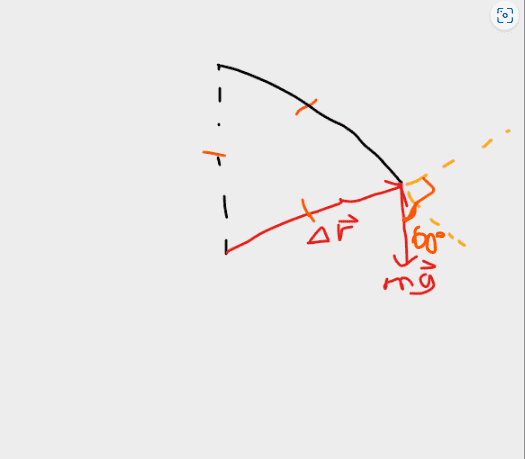Many thanks!

ChiralSuperfields
There are no 90 degrees angles, as you mistakenly show in your first diagram. How can you get 150?

Sorry, what do you mean there are no 90-degree angles?

I got 150 degrees since there is a right angle between the two dotted orange lines and a 60 degree angle between and the red mg line and dotted orange line.

Many thanks!

Homework Helper
Gold Member
2022 Award

Sorry, I did not mean the angle between the mg line at the dashed orange line at 90 degrees. It should be,
View attachment 322454

Many thanks!
Ok, but how do you get 60° between mg and the lower dashed orange line?
It would help if you were to draw it a bit more accurately.

•ChiralSuperfields
Homework Helper

Sorry, what do you mean there are no 90-degree angles?

I got 150 degrees since there is a right angle between the two dotted orange lines and a 60 degree angle between and the red mg line and dotted orange line.

Many thanks!
No, there is not. What is that dotted orange line? If you draw it perpendicular ot the direction of ##\Delta \vec{r} ## then the other angle is not 60 degrees. But you don't need that dotted line. Just draw the weight where ##\Delta \vec{r} ## starts.

•ChiralSuperfields
ChiralSuperfields
Ok, but how do you get 60° between mg and the lower dashed orange line?
It would help if you were to draw it a bit more accurately.

I think I got 60 degrees from the corresponding angles theorem.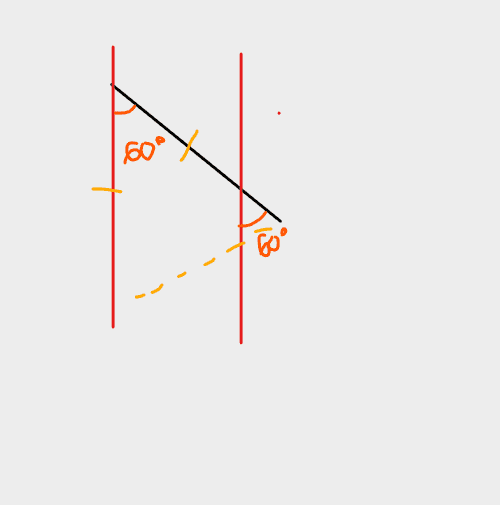Many thanks!

ChiralSuperfields
No, there is not. What is that dotted orange line? If you draw it perpendicular ot the direction of ##\Delta \vec{r} ## then the other angle is not 60 degrees. But you don't need that dotted line. Just draw the weight where ##\Delta \vec{r} ## starts.

Many thanks!

Homework Helper

I think I got 60 degrees from the corresponding angles theorem.
View attachment 322462

Many thanks!
That angle is 60 degrees but the extension of the black line is not along the dotted line that you show in the previous drawing. You see here that the black line is not at 90 degrees relative to this dotted orange line.

•ChiralSuperfields
Homework Helper
Gold Member
2022 Award
You keep bending lines.
Go back to your diagram in post #1, draw it accurately, and don't mark in right angles that you cannot prove are right angled.

•ChiralSuperfields
ChiralSuperfields
Thank you for your replies @nasu and @haruspex !

Here is the new diagram,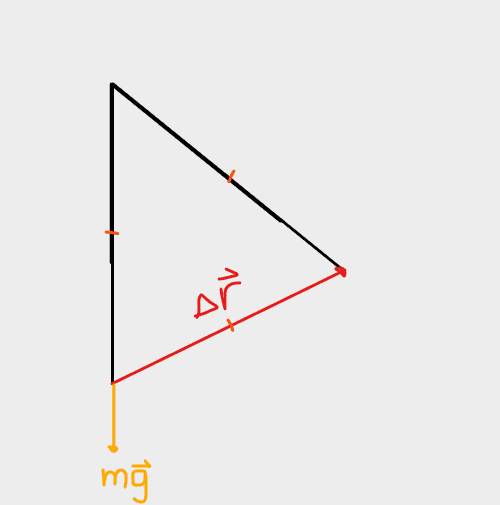How is it?

Many thanks!

Homework Helper
Gold Member
2022 Award
Thank you for your replies @nasu and @haruspex !

Here is the new diagram,
View attachment 322465
How is it?

Many thanks!
More accurate in some ways, but you have not marked in any angles, and you have moved the mg line over to the left.

•ChiralSuperfields
ChiralSuperfields
More accurate in some ways, but you have not marked in any angles, and you have moved the mg line over to the left.
Thank you for your reply @haruspex ! I moved the mg line to the left so I could see from exterior angle theorem that ##\theta = 120~degrees##.

What way were you going to find the angle?

I think I also found ##\vec {\Delta r}## vector correctly now.

To find it I split my equilateral triangle into half to form a right angle triangle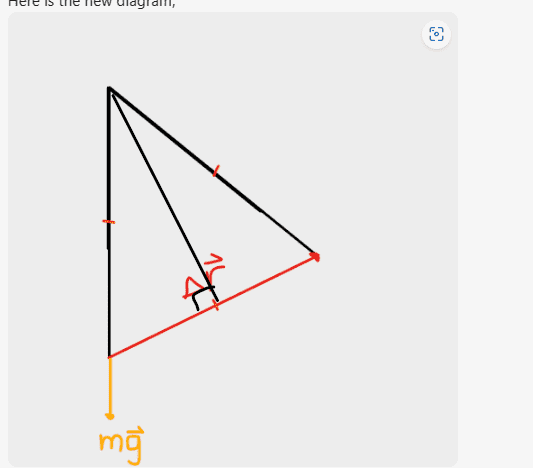I then found the opposite to be 6m so the total displacement must be 12m. But I guess I did not need to do that since the triangle has equal sides.

Many thanks!

Last edited:
Homework Helper
Gold Member
2022 Award
Thank you for your reply @haruspex ! I moved the mg line to the left so I could see from exterior angle theorem that ##\theta = 120~degrees##.

What way were you going to find the angle?

I think I also found ##\vec {\Delta r}## vector correctly now.

To find it I split my equilateral triangle into half to form a right angle triangle
View attachment 322466
I then found the opposite to be 6m so the total displacement must be 12m. But I guess I did not need to do that since the triangle has equal sides.

Many thanks!
Ok, so what is the angle between the force mg and the displacement ##\vec r##?

•ChiralSuperfields
Homework Helper
"Equilateral" means all sides are the same size (equal sides). You don't need to split it and to form right angle triangles. You seem to have a tendency to pick the most complicated ways to solve things., 😃

•ChiralSuperfields
Homework Helper
Gold Member
2022 Award
Hi @Callumnc1. I’d like to mention an alternative method.

Do you know how to find (changes in) gravitational potential energy? If so, you can use:

Work done by gravity = - (change in gravitational potential energy)

It’s well worth thinking about why the above method is equivalent to the ‘dot product’ method.

•ChiralSuperfields, Lnewqban and Chestermiller
Homework Helper
Gold Member
Thank you for your replies @haruspex and @Lnewqban!
...
F is the force of gravity acting on spider man and W is the work done by the force of gravity on the spider man.
How are those two forces different?
Acting only vertically, weight can only resist vertical displacement between lowest and highest points.
The muscular energy from Spiderman is the only cause of the horizontal displacement (r→), about which the problem is not asking.
Re-visit post #3 and focus only on pure vertical work.

•ChiralSuperfields